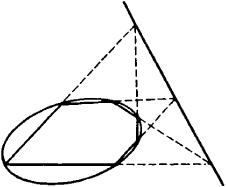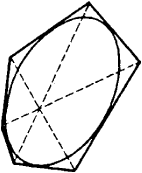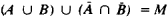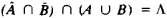# Duality, Principle of

The following article is from The Great Soviet Encyclopedia (1979). It might be outdated or ideologically biased.

## Duality, Principle of

a principle formulated in some branches of mathematics and consisting in the fact that to each true statement of the branch in question corresponds a dual statement which may be derived from the first by substituting the concepts entering into it with other, so-called, dual concepts.

(1) The principle of duality is formulated in plane projective geometry. In this case, the dual concepts are, for example, “point” and “straight line”; “the point lies on the straight line” and “the straight line passes through the point.” A dual proposition may be formulated for each axiom of plane projective geometry; this proposition may be proved using the same axioms (this is the basis for the principle of duality in plane projective geometry). Dual statements in plane projective geometry are the well-known theorems of Pascal and Brianchon. The first theorem claims that in every hexagon inscribed within a curve of the second order, the points of intersection of opposite sides lie on the same straight line (Figure 1). The second theorem asserts that in every hexagon circumscribed around a curve of the second order, the straight lines connecting the opposite vertices intersect at the same point (Figure 2).Figure 1Figure 2

(2) Principle of duality in the abstract theory of sets. Let there be a set M. Let us also examine the system of all of its subsets A, B, C, and so on. The following proposition is valid: if the theorem of the subsets of set M, which is formulated only in terms of the operations of union, intersection, and complement, is valid, then the theorem that is obtained on the basis of the first theorem by means of replacing the operations of union and intersection by the operations of intersection and union, the empty set Λ by the entire set M, and the set M by the empty set Λ is also valid. In this case, the complement of the union is replaced by the intersection of the complements, and the complement of the intersection is replaced by the union of the complements.

Example l. To the valid relationship

(AB) ∩ C = (AC) ∩ (BC)

corresponds the dual relationship (also valid)

(AB)C = (AC) ∩ (BC)

Example 2. To the valid relationshipcorresponds the dual relationship (also valid)where Ā, B are the complements of sets A, B in set M, A ∪ B is the union of sets A and B, and A ∩ B is their intersection.

(3) The principle of duality also occurs in mathematical logic (in propositional calculus and in predicate calculus).

### REFERENCES

Efimov, N. V. Vysshaia geomeiriia, 4th ed. Moscow, 1961.
Aleksandrov, P. S. Vvedenieν obshchuiu teoriiu mnozhestv i funktsii. Moscow-Leningrad. 1948.
Hilbert, D., and W. Ackerman. Osnovy teoreticheskoi logiki. Moscow. 1947. (Translated from German.)